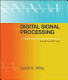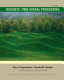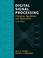### Digital Signal Processing

#### Course Description

Digital signal processing theory coupled with significant advances in digital computers enabled development of completely new electronic systems with wide range of applications. Signal processing is performed by a computer program on time samples of the input signals. Course covers the following topics: time discrete signals and Fourier transformation; introduction to DSP and digital filters; continuous time-domain filters; design of Finite Impulse Response (FIR) filters; optimal FIR filters; design of Infinite Impulse Response (IIR) filters, frequency transformations in Z domain; block and sample-by-sample processing; FIR and IIR filter structures, quantization of filter coefficients; Fast Fourier Transform (FFT), multirate signal processing; introduction to digital speech and image processing.

#### General Competencies

Student acquires fundamental theoretical knowledge of digital signal processing, of signal processing algorithms and methods, as well as basic skills related to the implementation and application of these methods.

#### Learning Outcomes

1. explain the significance of digital signal processing (DSP) in the fields of computing, electronic, automatic control, telecommunications and multi-media technology
2. interpret fundamental concepts such as 'linearity', 'time-invariance', 'impulse response', 'convolution', 'frequency response', 'z-transforms' and the 'discrete time Fourier transform', as applied to DSP
3. design ‘FIR type’ and ‘IIR type’ digital filters
4. compare and evaluate properties of designed ‘FIR type’ or ‘IIR type’ digital filters
5. analyze and implement discrete time and digital systems using Block Diagrams, Difference Equations and Z-Transforms
6. develop and implement FFT algorithms
7. interpret multirate signal processing

#### Forms of Teaching

Lectures

Lectures are held once weekly in a three hour session.

Exams

Knowledge assessments include homework, midterm exam, and both written and oral final exam.

Laboratory Work

Laboratory sessions are part of separate courses. They introduce students to practical problems related to digital signal processing.

Consultations

Lecturers in charge:

Lecturers:

E-learning

Students have at their disposal a Moodle repository with additional learning and automatized knowledge assessment materials.

#### Grading Method

Continuous Assessment Exam
Type Threshold Percent of Grade Threshold Percent of Grade
Homeworks 0 % 6 % 0 % 0 %
Mid Term Exam: Written 0 % 30 % 0 %
Final Exam: Written 0 % 40 %
Final Exam: Oral 24 %
Exam: Written 50 % 50 %
Exam: Oral 50 %
##### Comment:

Mandatory prerequisites for oral exams are achieving at least 50% of points on midterm and final exam combined, or on the written part of a regular exam. A minimum of 6 points is required to pass the final oral exam. A minimum of 50% of total oral exam points is required to pass the regular oral exam.

#### Week by Week Schedule

1. Introduction to digital signal processing, advantages and problems, filter specification. Examples of simple finite and infinite impulse response filters
2. Frequency domain representation of time discrete signals, aperiodic and periodic signals, digital processing of continuous time signals, sampling, antialiasing filtration
3. Reconstruction of the continuous time domain signal from its samples, ideal interpolation, real interpolators, spectrum sampling and reconstruction, Discrete Fourier Transform (DFT)
4. Fast Fourier Transform, FFT, divide and conquer method, decimation in time domain
5. Radix 2 FFT method, FFT butterfly, bit-reversed algorithm for sample addressing, computational complexity, RE2FFT algorithm, FFT based fast convolution
6. Filter transfer function, magnitude and phase response, group delay, introduction to digital filter design
7. Block based signal processing, sample by sample processing, convolution sum, direct implementation of linear convolution. Block based convolution, overlap-add method, periodic and circular convolution
8. Mitdterm exam
9. Design example of a FIR filter. Moving average filter. Classification of nonrecursive filters based on symmetricity. Gibbs phenomenon. FIR filter design using time-window method.
10. Adaptive time-window functions (Dolph-Chebyshev, Kaiser). Optimal FIR filter design, equiripple criterion (min-max), goal and spectral weighting function; numerical optimization procedures, Parks McClellan algorithm. Frequencies corresponding to approximation error extremes, Remez exchange algorithm, examples of optimal FIR filter design in Matlab.
11. Design of infinite impulse response filters by transforming continuous time domain filters to discrete domain, equivalent impulse response transformation, sampling and frequency response aliasing, relation of poles in S and Z domain, design procedure.
12. Bilinear transformation, properties, frequency mapping between continuous and discrete time domain frequencies, prewarping of filter specifications.
13. Frequency transformations in Z domain. Transformation of low-pass filter to a high-pass, band-pass, band-stop or low-pass with a new cutoff frequency. All-pass function of the transformed Z variable, nonlinear frequency mapping, computation of the cutoff frequencies of the transformed filters.
14. FIR and IIR filter structures, transformation of the signal flow graph, transposed structure, linear phase FIR structures, polyphase FIR structure. IIR filter structures, direct form type I and II, transposed direct form II, cascade and parallel IIR structures. Quantization of filter coefficients, sensitivity analysis, quantization of second order section, possible pole/zero locations.
15. Final exam

#### Study Programmes

##### University graduate
Computer Engineering (profile)
Recommended elective courses (3. semester)
Electronic and Computer Engineering (profile)
Theoretical Course (1. semester)
Information Processing (profile)
Theoretical Course (1. semester)
Wireless Technologies (profile)
Theoretical Course (1. semester)

#### LiteratureS. K. Mitra (2010.), DIGITAL SIGNAL PROCESSING: a Computer-Based Approach McGraw-Hill Co. Inc. 1998, McGraw-Hill Higher EducationA. V. Oppenheim, R. W.Schafer (2009.), Discrete-Time Signal Processing, Prentice Hall PTRJohn G. Proakis, Dimitris G. Manolakis (2006.), Digital Signal Processing, Prentice Hall
D. Petrinović, D. Petrinović, R. Bregović, H. Babić. i B. Jeren (2003.), Digitalna obradba signala - Upute za laboratorijske vježbe, FER-ZESOI - elektroničko izdanje

#### General

ID 34397
Winter semester
5 ECTS
L0 English Level
L1 e-Learning
45 Lectures
0 Exercises
0 Laboratory exercises
0 Project laboratory

87 Excellent
75 Very Good
64 Good
51 Acceptable